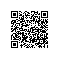# linux shell 字符串操作（长度，查找，替换）详解

${var} 变量var的值, 与$var相同

${var-DEFAULT} 如果var没有被声明, 那么就以$DEFAULT作为其值 *
${var:-DEFAULT} 如果var没有被声明, 或者其值为空, 那么就以$DEFAULT作为其值 *

${var=DEFAULT} 如果var没有被声明, 那么就以$DEFAULT作为其值 *
${var:=DEFAULT} 如果var没有被声明, 或者其值为空, 那么就以$DEFAULT作为其值 *

${var+OTHER} 如果var声明了, 那么其值就是$OTHER, 否则就为null字符串
${var:+OTHER} 如果var被设置了, 那么其值就是$OTHER, 否则就为null字符串

${var?ERR_MSG} 如果var没被声明, 那么就打印$ERR_MSG *
${var:?ERR_MSG} 如果var没被设置, 那么就打印$ERR_MSG *

${!varprefix*} 匹配之前所有以varprefix开头进行声明的变量${!varprefix@} 匹配之前所有以varprefix开头进行声明的变量

[chengmo@localhost ~]$echo${!v@}
var1 var2 var3
[chengmo@localhost ~]$echo${!v*}
var1 var2 var3

${!varprefix*}与${!varprefix@}相似，可以通过变量名前缀字符，搜索已经定义的变量,无论是否为空值。

${#string}$string的长度

${string:position} 在$string中, 从位置$position开始提取子串${string:position:length} 在$string中, 从位置$position开始提取长度为$length的子串${string#substring} 从变量$string的开头, 删除最短匹配$substring的子串
${string##substring} 从变量$string的开头, 删除最长匹配$substring的子串${string%substring} 从变量$string的结尾, 删除最短匹配$substring的子串
${string%%substring} 从变量$string的结尾, 删除最长匹配$substring的子串${string/substring/replacement} 使用$replacement, 来代替第一个匹配的$substring
${string//substring/replacement} 使用$replacement, 代替所有匹配的$substring${string/#substring/replacement} 如果$string的前缀匹配$substring, 那么就用$replacement来代替匹配到的$substring
${string/%substring/replacement} 如果$string的后缀匹配$substring, 那么就用$replacement来代替匹配到的$substring 说明："*$substring”可以是一个正则表达式.

1.长度

[web97@salewell97 ~]$test='I love china' [web97@salewell97 ~]$ echo ${#test} 12${#变量名}得到字符串长度

2.截取字串

[chengmo@localhost ~]$test='I love china' [chengmo@localhost ~]$ echo ${test:5} e china [chengmo@localhost ~]$ echo ${test:5:10} e china${变量名:起始:长度}得到子字符串

3.字符串删除

[chengmo@localhost ~]$test='c:/windows/boot.ini' [chengmo@localhost ~]$ echo ${test#/} c:/windows/boot.ini [chengmo@localhost ~]$ echo ${test#*/} windows/boot.ini [chengmo@localhost ~]$ echo ${test##*/} boot.ini [chengmo@localhost ~]$ echo ${test%/*} c:/windows [chengmo@localhost ~]$ echo ${test%%/*}${变量名#substring正则表达式}从字符串开头开始配备substring,删除匹配上的表达式。

${变量名%substring正则表达式}从字符串结尾开始配备substring,删除匹配上的表达式。 注意：${test##*/},${test%/*} 分别是得到文件名，或者目录地址最简单方法。 4.字符串替换 [chengmo@localhost ~]$ test='c:/windows/boot.ini'
[chengmo@localhost ~]$echo${test/\//\\}
c:\windows/boot.ini
[chengmo@localhost ~]$echo${test//\//\\}
c:\windows\boot.ini

${变量/查找/替换值} 一个“/”表示替换第一个，”//”表示替换所有,当查找中出现了：”/”请加转义符”\/”表示。 三、性能比较 在shell中，通过awk,sed,expr 等都可以实现，字符串上述操作。下面我们进行性能比较。 [chengmo@localhost ~]$ test='c:/windows/boot.ini'
[chengmo@localhost ~]$time for i in$(seq 10000);do a=${#test};done; real 0m0.173s user 0m0.139s sys 0m0.004s [chengmo@localhost ~]$ time for i in $(seq 10000);do a=$(expr length \$test);done;

real    0m9.734s
user    0m1.628s使用钉钉扫一扫加入圈子
+ 订阅